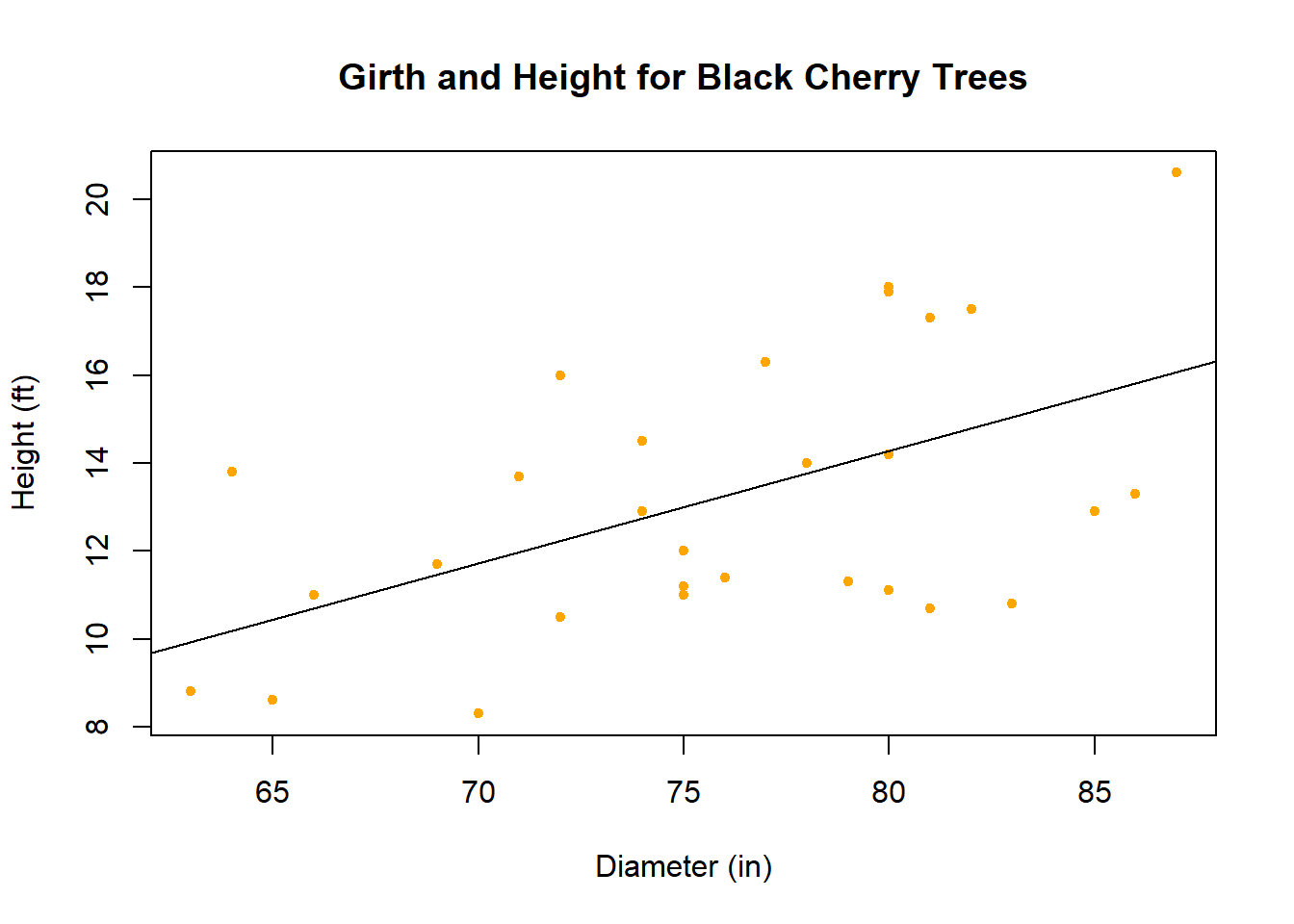## Black Cherry Trees

### Background

Data was collected on the girth, height, and volume of timber in 31 felled black cherry trees. It seems reasonable to assume that the bigger the girth of the trees, the taller it would be thus greater height. Data was collected both to see if this was true or not, and to estimate the relationship between girth and speed. Note that girth is the diameter of the tree (in inches) measured at 4 ft. 6 in. above the ground.

### Analysis

The hypotheses for the study concerns the slope. If the slope is zero, then there is not a meaningful relationship between the girth and height of the black cherry trees.

$H_0: \beta_1 = 0$ $H_a: \beta_1 \neq 0$

The scatterplot below demonstates the observed relationship between girth and height of the trees.There does appear to be some evidence of a relationship. Assuming the relationship is linear, the equation of the fitted line shown in the plot above is

Y = -6.19 + 0.26 X

The equation shows that the coefficient for girth in inches is 0.26 ft. The coefficient indicates that for every additional inch in girth, you can expect height to increase by an average of 0.26 ft. Overall, we would reject the null hypothesis that the slope is 0 because the p-value is 0.002758.

Estimate Std. Error t value Pr(>|t|)
Height 0.2557 0.07816 3.272 0.002758
(Intercept) -6.188 5.96 -1.038 0.3077
Fitting linear model: Girth ~ Height
Observations Residual Std. Error $$R^2$$ Adjusted $$R^2$$
31 2.728 0.2697 0.2445

### Interpretation

The estimated value of the slope is 0.256 which shows that on average, the black cherry trees’ height is three quarters more than its girth. More specifically, we expect the black cherry trees to stand at 0.256 times what the girth of the tree is. A 95% confidence interval for the true slope is (0.0958955, 0.4155988) which is useful for demonstrating that the value of 1 is not in the interval, which value would imply that the girth and height of the tree are directly proportional.

This means that as the black cherry trees ages and grows fatter around the circumference of the trunk, the trees will grow taller. This is most likely due to the support that the tree needs as it grows in height to provide stability and balance.